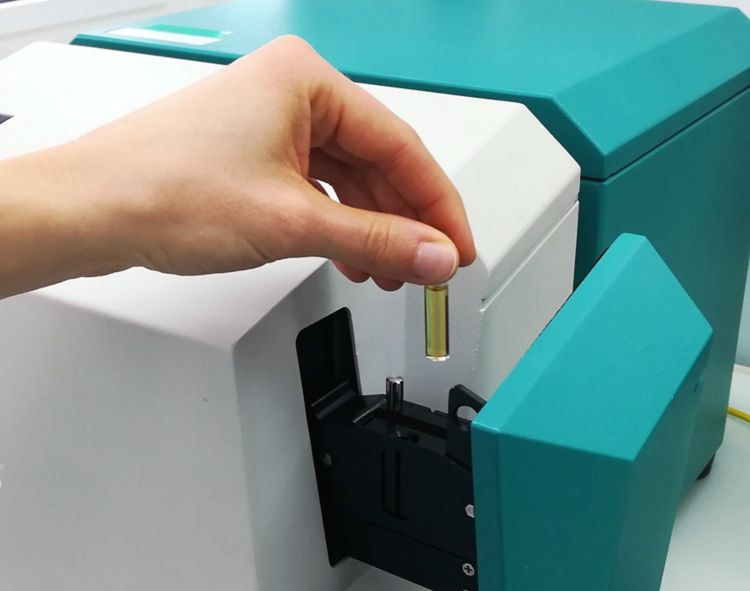Byli jste přesměrováni na vaši místní verzi požadované stránky

The cetane index (ASTM D613), flash point (ASTM D56), cold filter plug point (CFPP) (ASTM D6371), D95 (ISO 3405), and viscosity at 40 °C (ISO 3104) are key parameters to determine for diesel quality. The primary test methods are labor intensive and challenging due to the need to use different analytical methods.

This application note demonstrates that the XDS RapidLiquid Analyzer, operating in the visible and near-infrared spectral region (Vis-NIR) provides a cost-efficient and fast solution for the simultaneous determination of these key parameters in diesel. Vis-NIR spectroscopy allows for the analysis of diesel in less than one minute without sample preparation or using any chemical reagents.Figure 1. XDS RapidLiquid Analyzer with 8 mm disposable vial filled with a diesel sample.

Diesel samples were measured in transmission mode with a XDS RapidLiquid Analyzer (RLA) over the full wavelength range (400–2500 nm). The built-in temperature controlled sample chamber was set to 30 °C to provide a stable sample environment. For convenience reasons, disposable vials with a path length of 8 mm were used, which made a cleaning procedure obsolete. The Metrohm software package Vision Air Complete was used for data acquisition and prediction model development.

Table 1. Hardware and software equipment overview
Equipment Metrohm number
XDS RapidLiquid Analyzer 2.921.1410
Disposable vials, 8 mm diameter, transmission 6.7402.000
Vision Air 2.0 Complete 6.6072.208

The obtained Vis-NIR spectra (Figure 2) were used to create prediction models for the determination of key diesel parameters. The quality of the prediction models was evaluated using correlation diagrams, which display the correlation between Vis-NIR prediction and primary method values. The respective figures of merit (FOM) display the expected precision of a prediction during routine analysis.

Figure 2. Selection of diesel Vis-NIR spectra obtained using a XDS RapidLiquid Analyzer and 8 mm disposable vials. For display reasons a spectra offset was applied.

### Result cetane index

Figure 3. Correlation diagram for the prediction of the cetane index using a XDS RapidLiquid Analyzer. The cetane index lab value was calculated based on the density and distillation range.
Table 2. Figures of merit for the prediction of the cetane index using a XDS RapidLiquid Analyzer.
Figures of merit Value
R2 0.991
Standard error of calibration 0.093
Standard error of cross-validation 0.143

### Result flash point

Figure 4. Correlation diagram for the prediction of the flash point using a XDS RapidLiquid Analyzer. The flash point lab value was evaluated using a dedicated flash point analyzer.
Table 3. Figures of merit for the prediction of the flash point using a XDS RapidLiquid Analyzer.
Figures of merit Value
R2 0.977
Standard error of calibration 2.22 °C
Standard error of cross-validation 2.50 °C

### Result CFPP

Figure 5. Correlation diagram for the prediction of CFPP using a XDS RapidLiquid Analyzer. The lab value was evaluated using flow meters.
Table 4. Figures of merit for the prediction of CFPP using a XDS RapidLiquid Analyzer.
Figures of merit Value
R2 0.973
Standard error of calibration 1.99 °C
Standard error of cross-validation 2.24 °C

### Result D95

Figure 6. Correlation diagram for the prediction of D95 value using a XDS RapidLiquid Analyzer. The D95 lab value was evaluated using distillation.
Table 5. Figures of merit for the prediction of D95 using a XDS RapidLiquid Analyzer.
Figures of merit Value
R2 0.861
Standard error of calibration 3.07 °C
Standard error of cross-validation
3.47 °C

### Result viscosity

Figure 7. Correlation diagram for the prediction of the viscosity at 40 °C using a XDS RapidLiquid Analyzer. The viscosity lab value was evaluated using viscometry.
Table 6. Figures of merit for the prediction of the viscosity at 40 °C using a XDS RapidLiquid Analyzer.
Figures of merit Value
R2 0.983
Standard error of calibration 0.038 cSt
Standard error of cross-validation 0.047 cSt

This study demonstrates the feasibility of NIR spectroscopy for the analysis of the key parameters of diesel samples. In comparison to the standard methods, no sample preparation is needed. Samples are analyzed as they are, which allows for simple operation when using NIR spectroscopy.

To view the information for all key parameters and to get the latest information, please check out our precalibrations:

Pre-calibrations